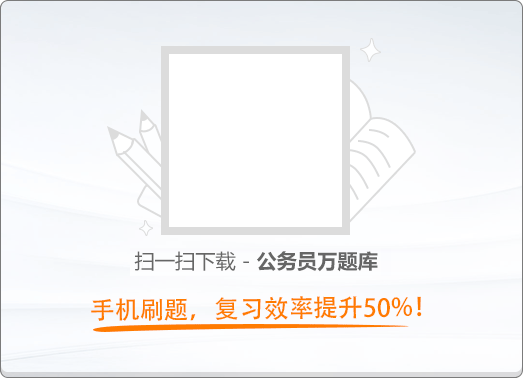120:002013年国家公务员考试《行测》真题

1
(单项选择题)

《国家“十二五”时期文化改革发展规划纲要》提出要加大政府对文化事业建设的投入力度。下列属于政府投入保障政策的是（　）。

• A.

支持文化企业在海外投资、投标、营销、参展和宣传等活动

• B.

继续完善文化市场的准入政策，吸引社会资本投资文化产业

• C.

文化内容创意生产、非物质文化遗产项目经营享受税收优惠

• D.

通过政府购买服务的方式，鼓励社会力量提供公共文化产品

• A
• B
• C
• D

2
(单项选择题)

• A.

古田会议：解决新型人民军队建设问题

• B.

遵义会议：纠正“左倾”的军事路线

• C.

洛川会议：决定北上抗日的总方针

• D.

瓦窑堡会议：确定建立抗日民族统一战线的方针政策

• A
• B
• C
• D

3
(单项选择题)

• A.

河西山冈万丈高，河东河北高梁熟了

• B.

我们生长在这里，每一寸土地都是我们自己的

• C.

每个人被迫着发出最后的吼声

• D.

宽广美丽的土地，是我们亲爱的家乡

• A
• B
• C
• D

4
(单项选择题)

• A.

赤字率——财政风险

• B.

恩格尔系数——收入分配差距

• C.

基尼系数——居民生活水平

• D.

生产者物价指数——货币供应量

• A
• B
• C
• D

5
(单项选择题)

• A.

最低工资保障

• B.

最低生活保障

• C.

税收

• D.

社会保险

• A
• B
• C
• D

6
(单项选择题)

①北师覆没威海卫，签订条约在马关

②鸦片带来民族难，销烟虎门海滩前

③武装起义占三镇，武昌汉口和汉阳

• A.

①③②

• B.

②③①

• C.

①②③

• D.

②①③

• A
• B
• C
• D

7
(单项选择题)

①某食品厂生产腐竹时非法添加硼砂被当场查获，县工商局以证据确凿为由吊销该厂营业执照，不再另行举行听证会；

②县矿业公司将含镉的工业废渣倾倒入河，造成河水镉浓度超标，县环保局、县水利局分别决定对其罚款10万元和5万元；

③县卫生局接到群众举报某火锅店使用过期牛油，遂派一工作人员前住检查，对牛油进行查封、送检。

• A.

县工商局

• B.

县环保局

• C.

县水利局

• D.

县卫生局

• A
• B
• C
• D

8
(单项选择题)

• A.

春秋之治狱，论心定罪——自由裁量权

• B.

载于简牍谓之书，合而验之谓之契——合同

• C.

一兔走街，百人追之；积兔满市，过而不顾——物权

• D.

罪疑惟轻，功疑惟重，与其杀不辜，宁失不经——疑罪从无

• A
• B
• C
• D

9
(单项选择题)

• A.

某公立大学以其教学大楼产权作抵押向银行申请贷款

• B.

某乡镇企业以所属土地所有权作抵押向银行申请贷款

• C.

某农民以所属宅基地的使用权作抵押向银行申请贷款

• D.

某国有企业以所属土地使用权作抵押向银行申请贷款

• A
• B
• C
• D

10
(单项选择题)

“天下九塞，雁门为首”，下列诗词描写“雁门关”的是（　）。

• A.

黑云压城城欲摧，甲光向日金鳞开

• B.

出关去，往辽东，诏徙十万填新丰

• C.

长城饮马寒宵月，古戍盘雕大漠风

• D.

八百里分麾下炙，五十弦翻塞外声，沙场秋点兵

• A
• B
• C
• D

11
(单项选择题)

• A.

《桃花扇》——借离合之情，写兴亡之感

• B.

《石头记》——满纸荒唐言，一把辛酸泪

• C.

《儒林外史》——一部儒林，终之以琴，滔滔天下，谁是知音

• D.

《聊斋志异》——使神魔皆有人情，精魅亦通世故

• A
• B
• C
• D

12
(单项选择题)

• A.

人的唾液是由舌头分泌的

• B.

胆分泌胆汁不足，容易导致胆囊炎

• C.

食盐、鱼肝油可被消化道直接吸收

• D.

正常情况下，卵细胞在子宫内受精

• A
• B
• C
• D

13
(单项选择题)

• A.

辛味药：连翘、杏仁

• B.

甘味药：当归、人参

• C.

酸味药：陈皮、黄连

• D.

苦味药：黄柏、乌梅

• A
• B
• C
• D

14
(单项选择题)

• A.

雹、霜、雾

• B.

雨、雪、霜

• C.

雪、露、雾

• D.

雨、雪、雹

• A
• B
• C
• D

15
(单项选择题)

• A.

蓝藻→蕨类→裸子植物→被子植物

• B.

节肢动物→草履虫→软体动物→脊椎动物

• C.

细菌→腔肠动物→爬行类→鱼类

• D.

苔藓→绿藻斗菌类→双子叶植物

• A
• B
• C
• D

16
(单项选择题)

• A.

“海洋”

• B.

“风云”

• C.

“天绘”

• D.

“北斗”

• A
• B
• C
• D

17
(单项选择题)

《撒马拉斯颂歌》1958年被正式确定为现代奥林匹克运动会永久会歌，并改名《奥林匹克颂歌》。下列关于该会歌的表述错误的是（　）。

• A.

《奥林匹克颂歌》是首庄严悠扬的乐曲

• B.

开、闭幕式都要演唱《奥林匹克颂歌》

• C.

《撒马拉斯颂歌》改编自古罗马管弦乐

• D.

《撒马拉斯颂歌》出现时间早于五环旗

• A
• B
• C
• D

18
(单项选择题)

• A.

赤道→南回归线→赤道

• B.

赤道→北回归线→赤道

• C.

南回归线→赤道→北回归线

• D.

北回归线→赤道→南回归线

• A
• B
• C
• D

19
(单项选择题)• A.

A

• B.

B

• C.

C

• D.

D

• A
• B
• C
• D

20
(单项选择题)

• A.

人在屋里热得跳，稻在田里哈哈笑

• B.

麦苗盖上雪花被，来年枕着馍馍睡

• C.

立秋无雨样样有，立秋有雨收半秋

• D.

下秧太冷怕烂秧，小秧出水怕青霜

• A
• B
• C
• D